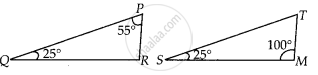# In ∆PQR and ∆MST, ∠P = 55°, ∠Q = 25°, ∠M = 100° and ∠S = 25°. Is ∆QPR ~ ∆TSM? Why? - Mathematics

Sum

In ∆PQR and ∆MST, ∠P = 55°, ∠Q = 25°, ∠M = 100° and ∠S = 25°. Is ∆QPR ~ ∆TSM? Why?

#### Solution

False

We know that, the sum of three angles of a triangle is 180°.

In ∆PQR, ∠P +∠Q +∠R = 180°

⇒ 55° + 25 ° + ∠R = 180°

⇒∠R = 180° – (55° + 25 °)

= 180° – 80° = 100°

In ∆TSM, ∠T + ∠S + ∠M = 180°

⇒ ∠T + ∠25° + 100° = 180°

⇒ ∠T = 180° – (25° + 100°) = 180° – 125° = 55°In ∆PQR and ∆TSM,

∠P = ∠T, ∠Q = ∠S and ∠R = ∠M

∴ ∠PQR = ∠TSM

[Since, all corresponding angles are equal]

Hence, ∆QPR is not similar to ∆TSM, since correct correspondence is P ↔ T, Q ↔ S and R ↔ M.

Concept: Similarity of Triangles
Is there an error in this question or solution?

#### APPEARS IN

NCERT Mathematics Exemplar Class 10
Chapter 6 Triangles
Exercise 6.2 | Q 5 | Page 64
Share